## The kidney

• Filter the blood
• Process starts with the glomeruli
• Also the site of many kidney diseases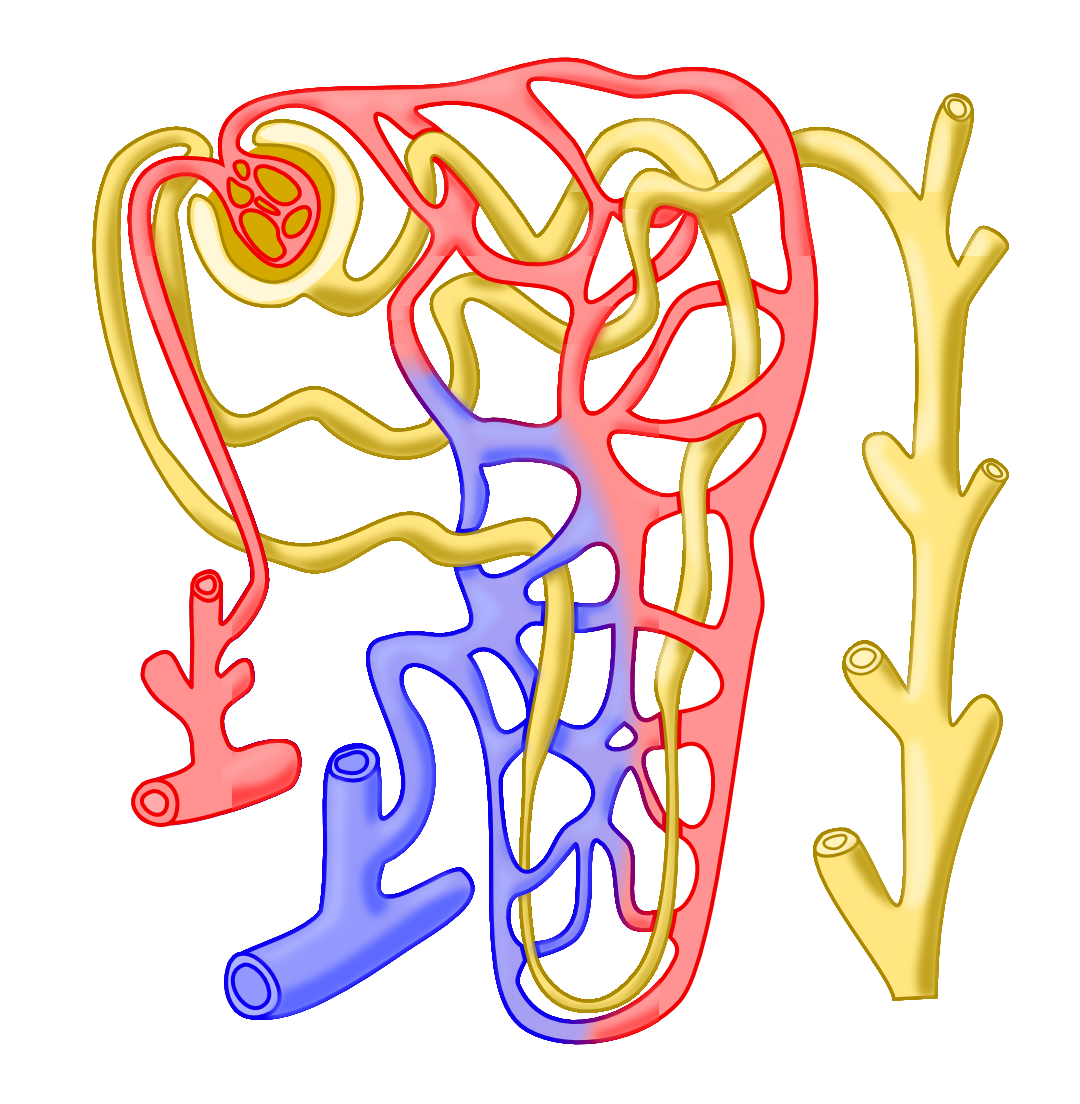## Glomeruli are undercounted

Pathologists consistently miss 50% of glomeruli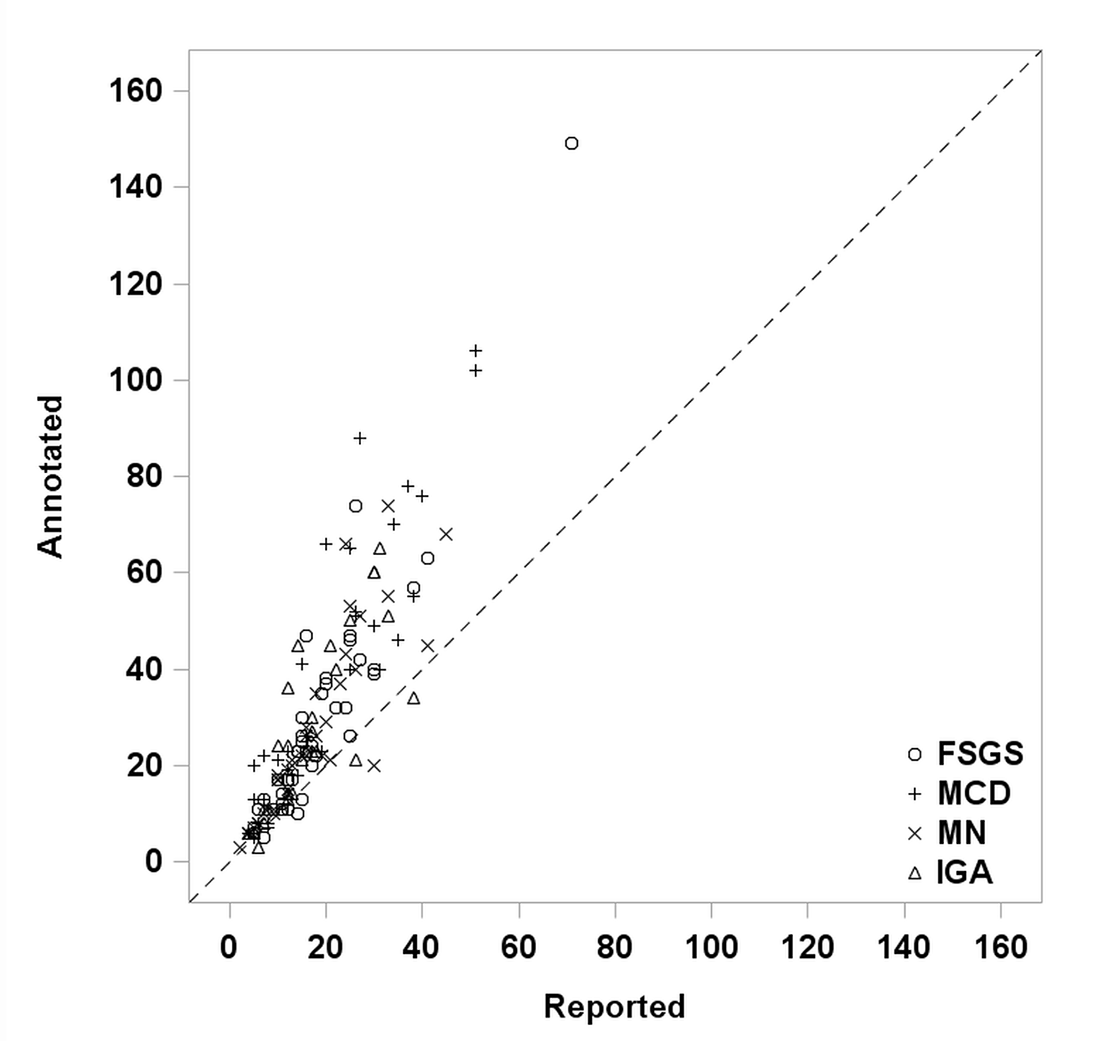### Simple classification model: performance baseline

 Positive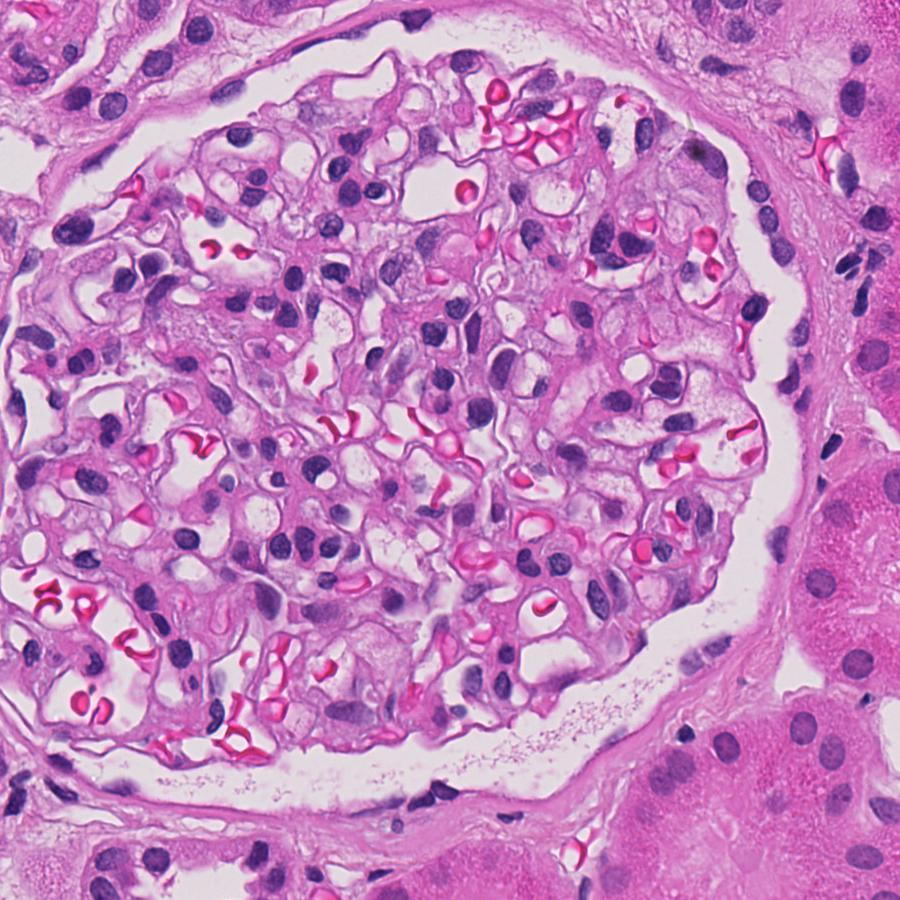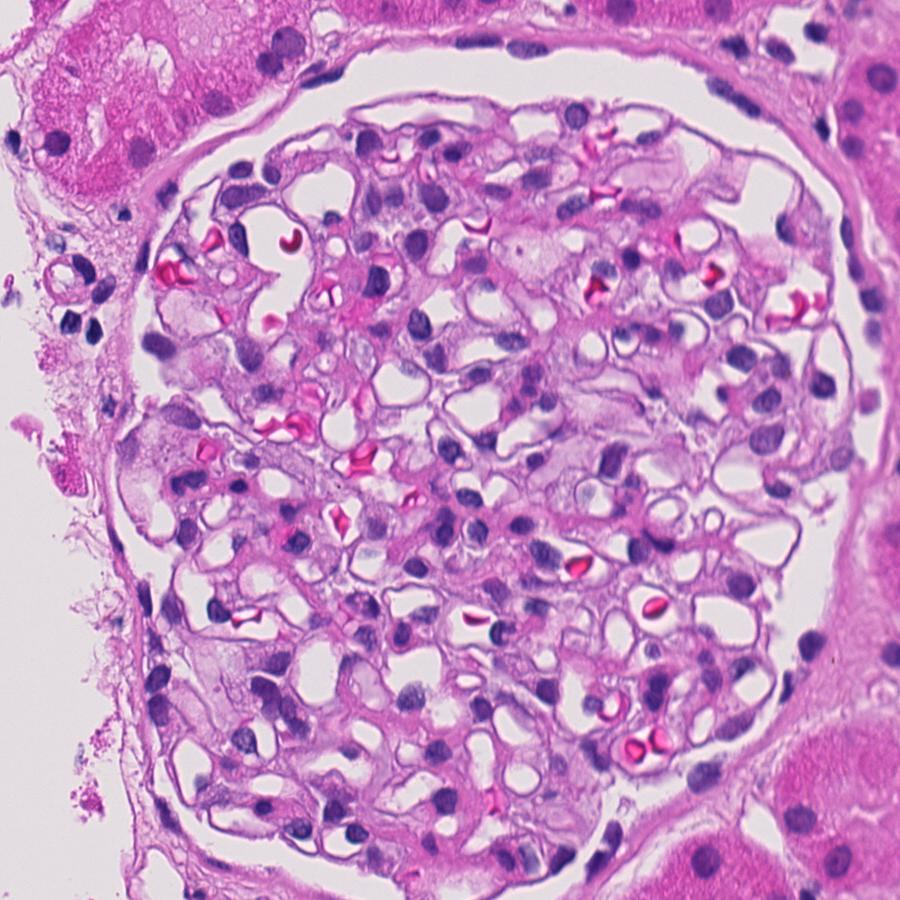Negative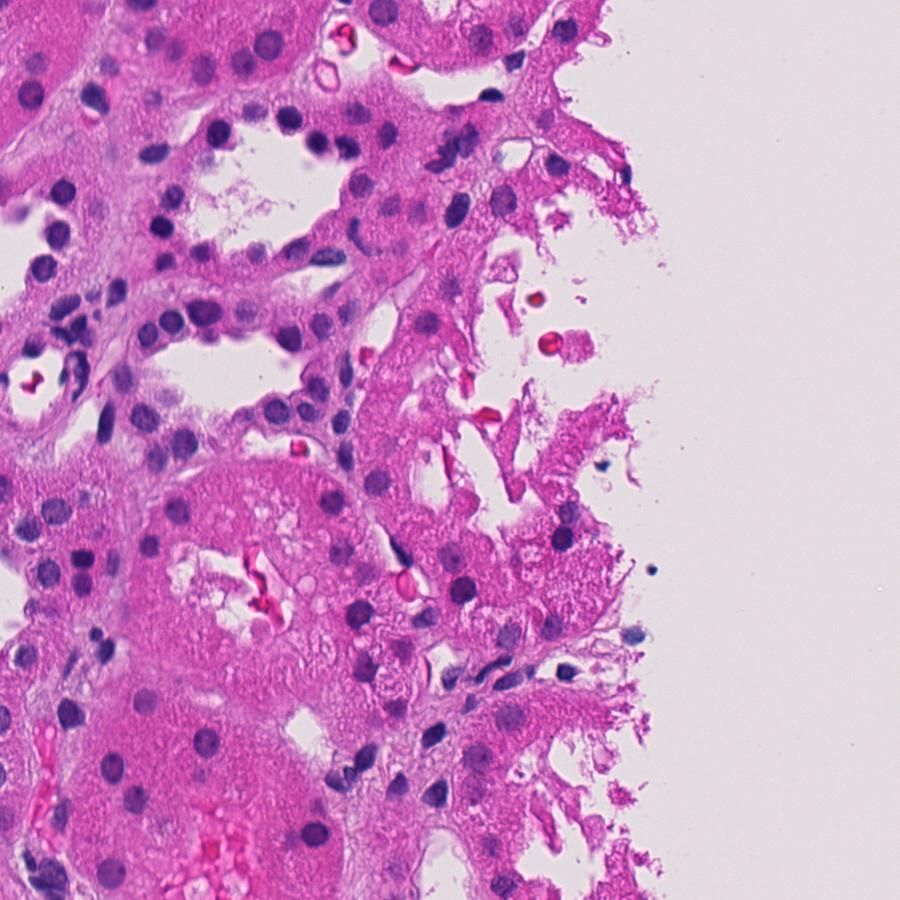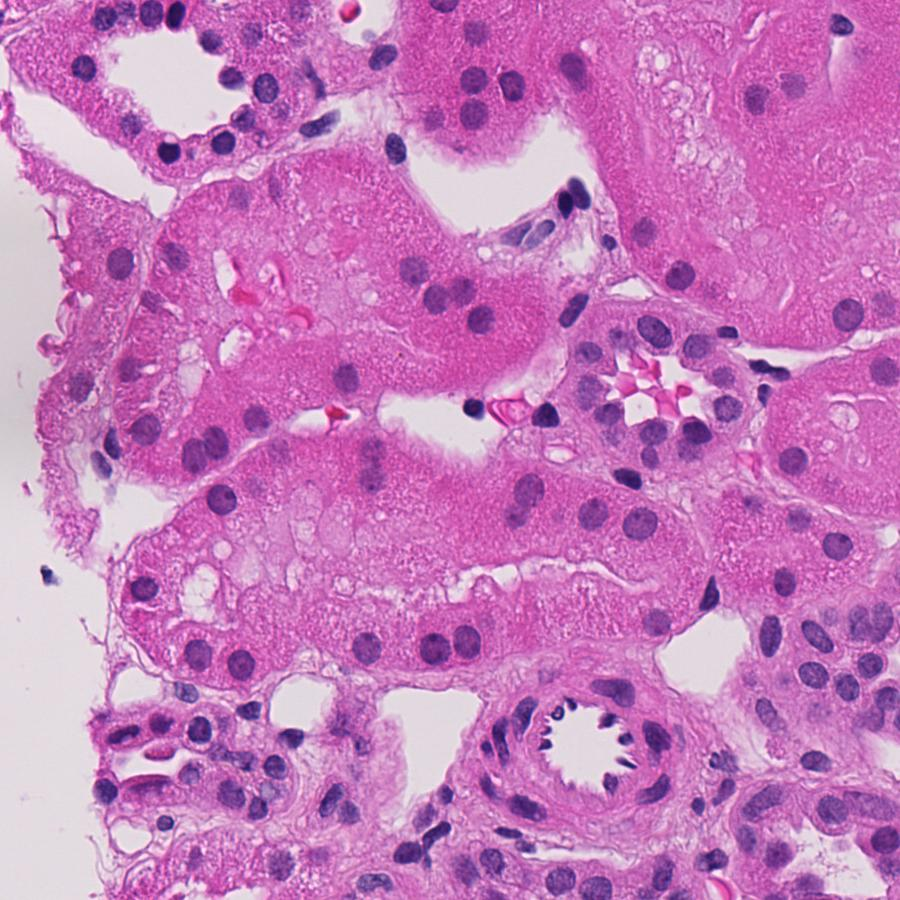### Simple classification model: performance baseline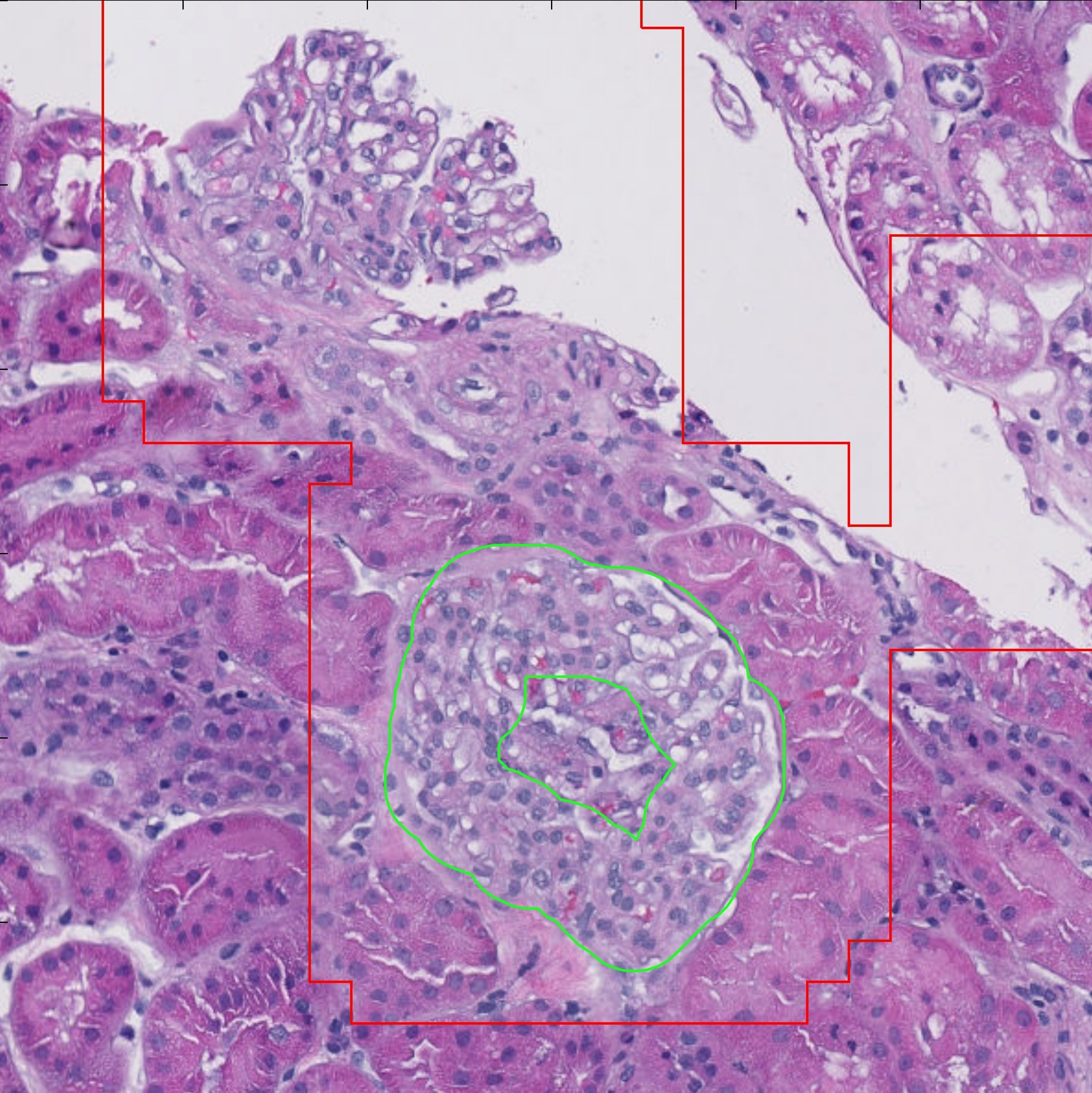### Segmentation

Is this pixel part of a glomerulus?

Fully convolutional neural networks appear useful

### Segmentation

Maxpooling to expand the perceptive field

Concatenate higher resolution features to refine boundary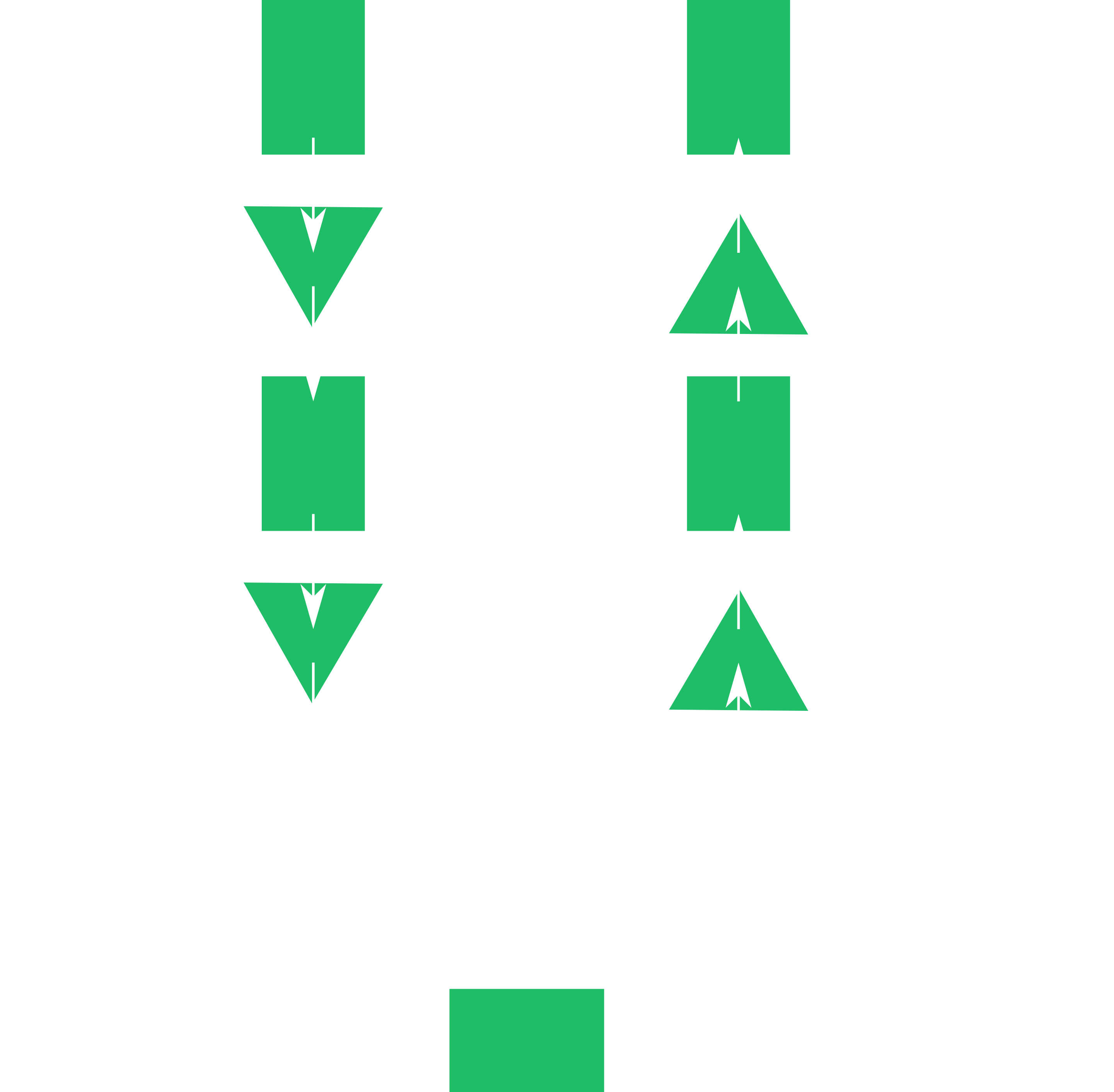## U-net model

``````
def unet_generator(input_shape=(1024,1024,3), num_classes=1, levels=7):
inputs = Input(shape=input_shape)

upsampling = []
connection = inputs
for i in range(levels):
down = Conv2D(8*2**i, (3, 3), padding='same')(connection)
down = BatchNormalization()(down)
down = Activation('relu')(down)
down = Conv2D(8*2**i, (3, 3), padding='same')(down)
down = BatchNormalization()(down)
down = Activation('relu')(down)
upsampling.append(down)
connection = MaxPooling2D((2, 2), strides=(2, 2))(down)

center = Conv2D(8*2**levels, (3, 3), padding='same')(connection)
center = BatchNormalization()(center)
center = Activation('relu')(center)
center = Conv2D(8*2**levels, (3, 3), padding='same')(center)
center = BatchNormalization()(center)
connection = Activation('relu')(center)

for i in range(levels-1, -1, -1):
up = UpSampling2D((2, 2))(connection)
up = concatenate([upsampling.pop(), up], axis=1)
up = Conv2D(8*2**i, (3, 3), padding='same')(up)
up = BatchNormalization()(up)
up = Activation('relu')(up)
up = Conv2D(8*2**i, (3, 3), padding='same')(up)
up = BatchNormalization()(up)
up = Activation('relu')(up)
up = Conv2D(8*2**i, (3, 3), padding='same')(up)
up = BatchNormalization()(up)
connection = Activation('relu')(up)

classify = Conv2D(num_classes, (1, 1), activation='sigmoid')(connection)

model = Model(inputs=inputs, outputs=classify)

model.compile(optimizer=RMSprop(lr=1e-3),
loss=bce_dice_loss,
metrics=[dice_coeff])

return model
``````